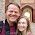## Thursday, October 31, 2013

### Tobit fitted values not "fitting" data

```* I was recently asked by a reader why it might be that the predicted values from
* a tobit regression might have a constant significantly below zero and many fitted
* values unrealistically below zero.

* Is that a problem?

* Let's do a simple simulation of what the Tobit might be doing:

clear
set obs 10000

gen x = rnormal()

gen y_true = -2 + x*2 + rnormal()*4

gen y_observed = y_true
replace y_observed = 0 if y_true < 0

hist y_ob```
```
```
```

tobit y_ob x, ll(0)

predict y_hat

graph twoway  (scatter y_hat y_true) (lfitci y_hat y_true)
```
```
* Not the best fit but, okay.

* So the take away. It is not inconsistent with the Tobit model at all that
* the constant and many fitted values may be significantly below zero.

* In a way, that is typically the result when it is most important to use a Tobit
* because you observe few values which are positive indicating that the underlying
* function is typically having many values fit below zero which have been censored.

* When you have the case in which the constant and many fitted values above zero,
* you have the least gains in terms of reducing bias from using a Tobit estimation
* method.

Formatted By Econometrics by Simulation
```

1.2.1.